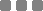PointSpot PackagesREMARK

1  The price is exclusive of 7% VAT

2  Member means the number of end user who got member card or reward point

3  Transaction means the number of SMS sent out when reward point, coupon, member card etc. were given to end user

Calculation Example of Business A using PREMIUM package at the end of the month

•  Members reached = 3,500 (The first 3,000 members are free of charge) Excess number of members = 500

•  Transactions used = 1,200 (The first 1,000 transactions are free of charge) Excess number of transactions = 200

Total fee for Business A at the end of the month

Monthly fee   PREMIUM Package = 1,490 THB

Member   Excess number of members  = 500 x 0.6  = 300 THB

Transaction   Excess number of transactions = 200 x 0.6  = 120 THB

Grand Total (1,490 + 300 + 120) + VAT 7%  = 2,044 THB